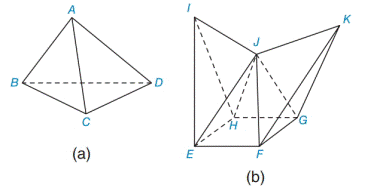Chapter 9.4, Problem 1E### Elementary Geometry for College St...

6th Edition
Daniel C. Alexander + 1 other
ISBN: 9781285195698

#### Solutions

Chapter
Section### Elementary Geometry for College St...

6th Edition
Daniel C. Alexander + 1 other
ISBN: 9781285195698
Textbook Problem
1 views

# Which of these two polyhedrons is concave? Note that the interior dihedral angle formed by the planes containing ∆EJF and ∆KJF is larger than 180 ° .To determine

To find:

The concave polyhedron.

Explanation

Approach:

1) A polygon is a two dimensional shape form with more than two straight lines.

2) A polyhedron is a three-dimensional shape made up of polygons as sides.

3) Each flat surface of a polyhedron is a polygon and is called a face. A polyhedron must have at least four faces.

4) The line segment where two faces of a polyhedron meet is called an edge.

5) The point where three or more edges of a polyhedron meet is called a vertex.

6) A dihedral angle is the angle between two faces with a common edge.

7) A polyhedron has dihedral angles between each of their sides because each side

of a polyhedron is a plane.

8) When a polyhedron is convex, each face determines a plane for which all remaining faces lie on same side of that plane...

### Still sussing out bartleby?

Check out a sample textbook solution.

See a sample solution

#### The Solution to Your Study Problems

Bartleby provides explanations to thousands of textbook problems written by our experts, many with advanced degrees!

Get Started

#### Solve the equations in Exercises 126. (x+1)3+(x+1)5=0

Finite Mathematics and Applied Calculus (MindTap Course List)

#### The difference between population variance and sample variance.

Statistics for The Behavioral Sciences (MindTap Course List)

#### In Exercises 1520, simplify the expression. 20. 81x5y1039xy23

Applied Calculus for the Managerial, Life, and Social Sciences: A Brief Approach

#### In Exercises 1-8, determine which of the matrices are regular. 

Finite Mathematics for the Managerial, Life, and Social Sciences